Abstract: 本文主要研究几个关于共享内存的例子，以此来了解共享内存的性质，为我们的核函数加速
Keywords: 行主序，列主序，填充与无填充，从线程索引体映射数据元素

# 共享内存的数据布局

• 方阵与矩阵数组
• 行主序与列主序
• 静态与动态共享内存的声明
• 文件范围与内核范围的共享内存
• 内存填充与无内存填充

1. 跨内存存储体映射数据元素
2. 从线程索引到共享内存偏移的映射

## 方形共享内存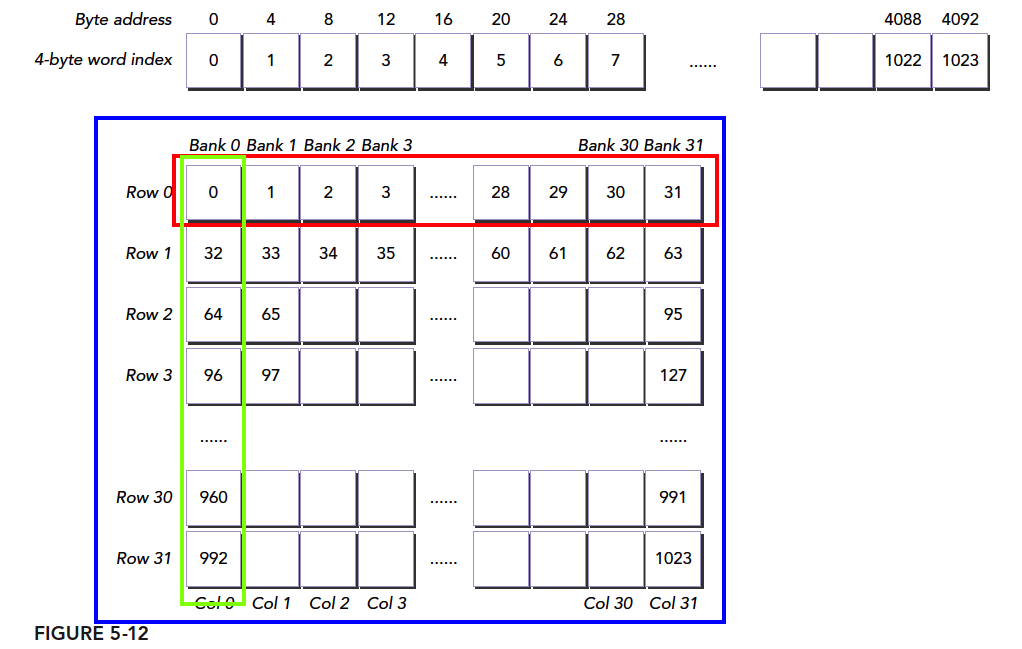### 行主序访问和列主序访问

• 将全局线程索引值存入二维共享内存
• 从共享内存中按照行主序读取这些值并存到全局内存中

• 定义一个共享内存，大小为 $32\times 32$
• 计算当前线程的全局位置的值idx
• 同步

1. 共享内存的写入
2. 共享内存的读取
3. 全局内存的写入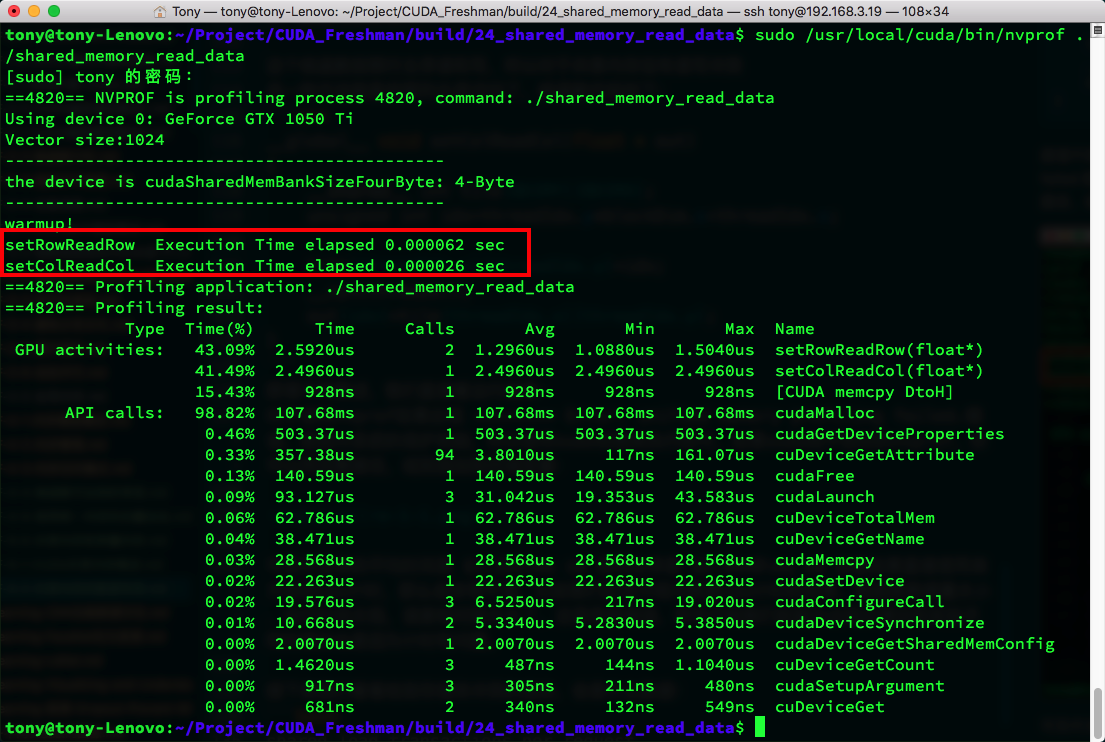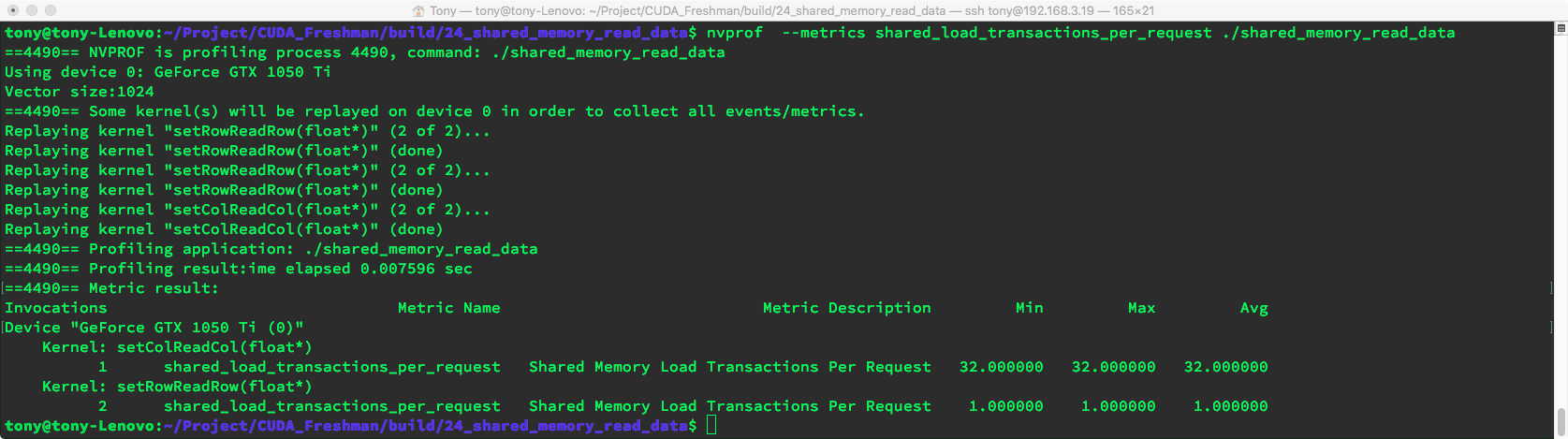• shared_store_transactions_per_request 结果：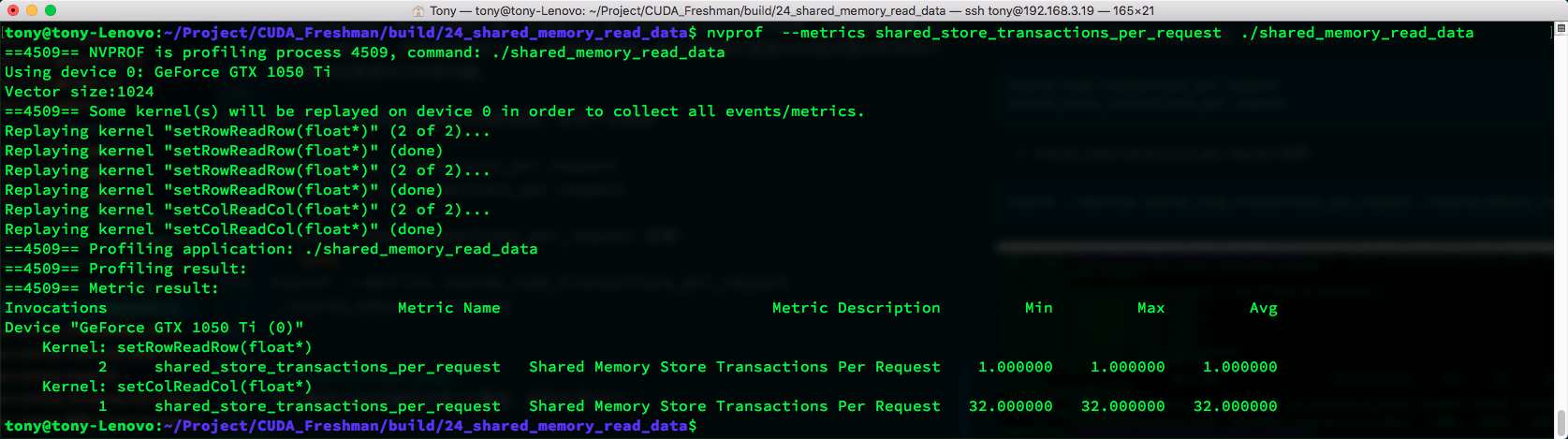### 按行主序写和按列主序读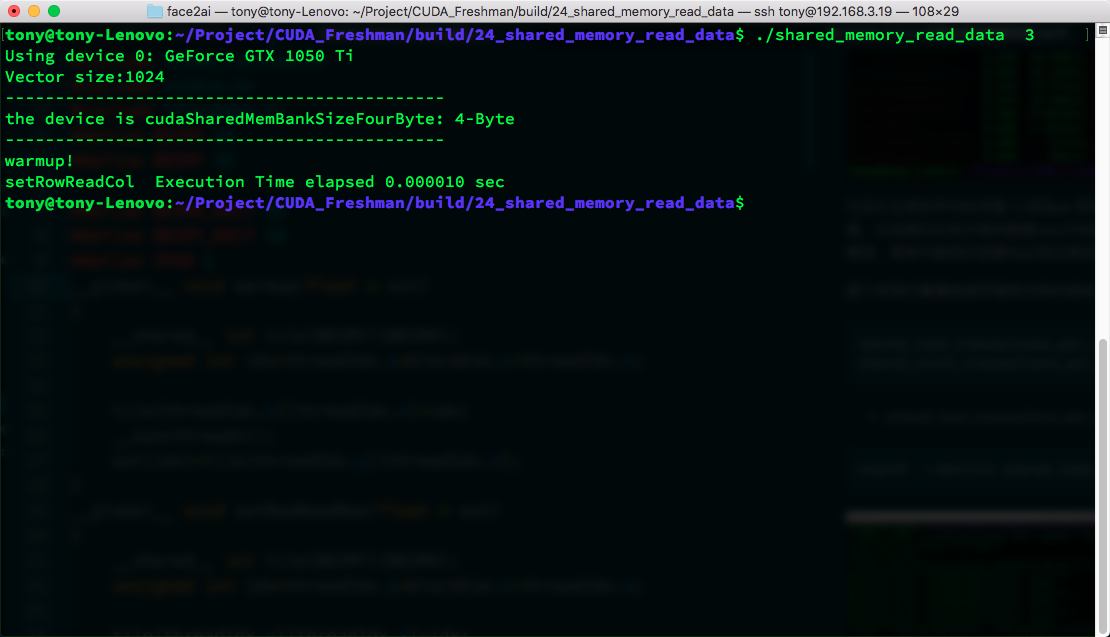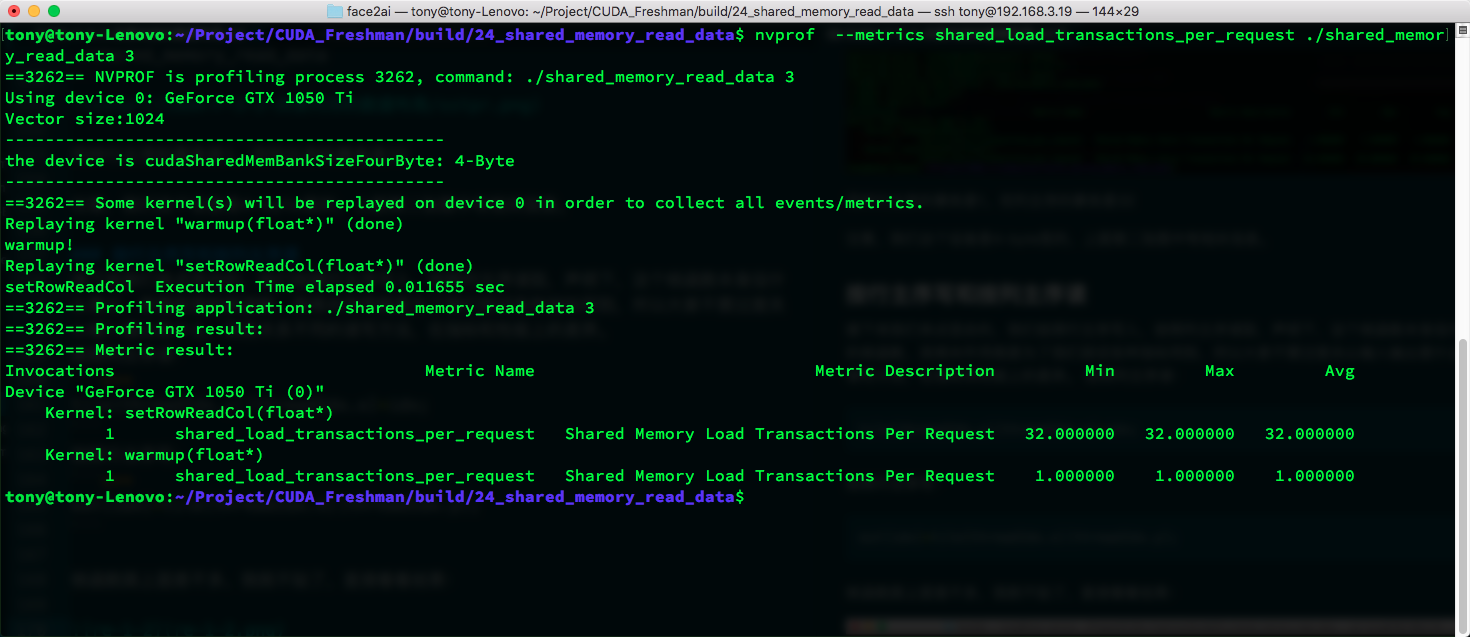shared_store_transactions_per_request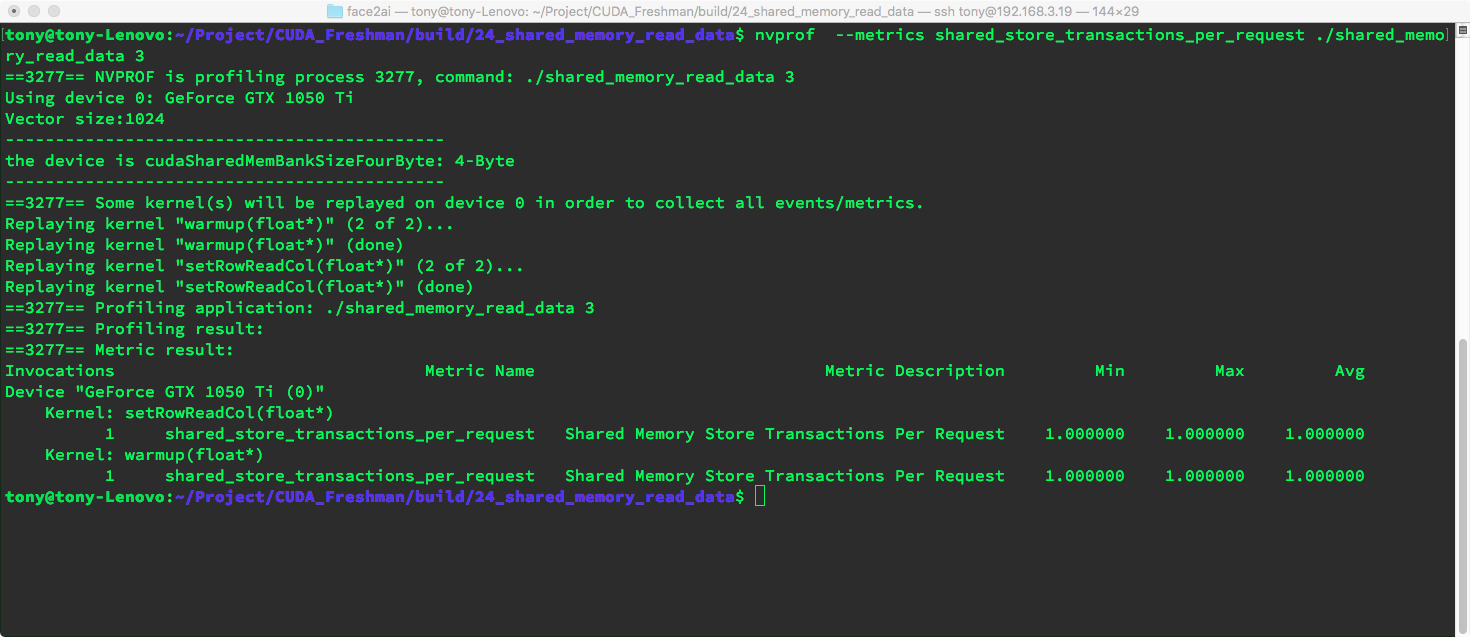### 动态共享内存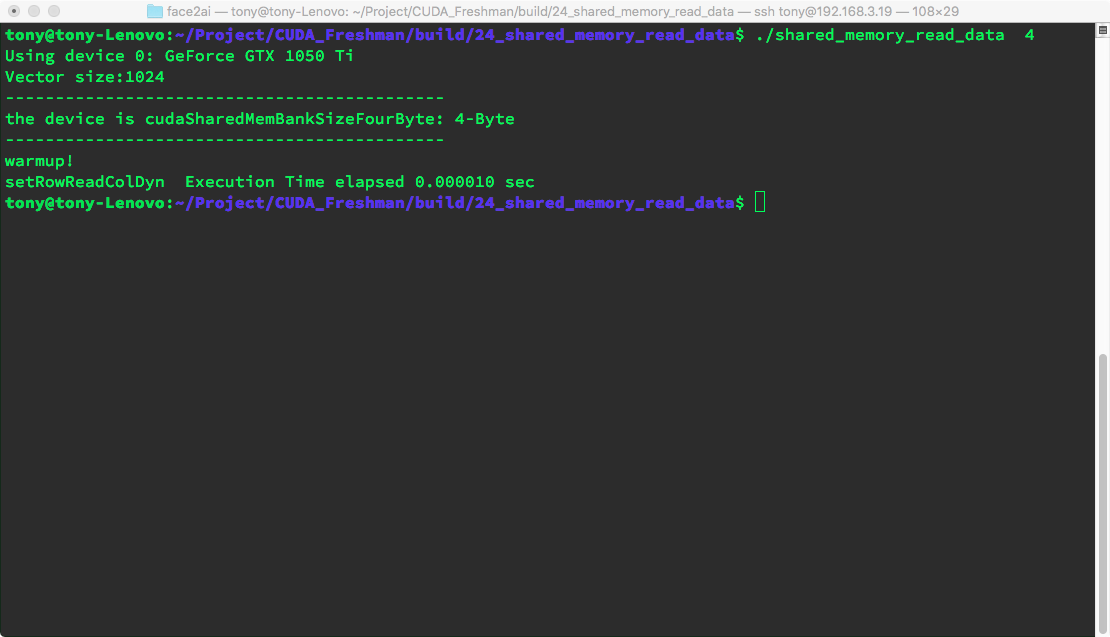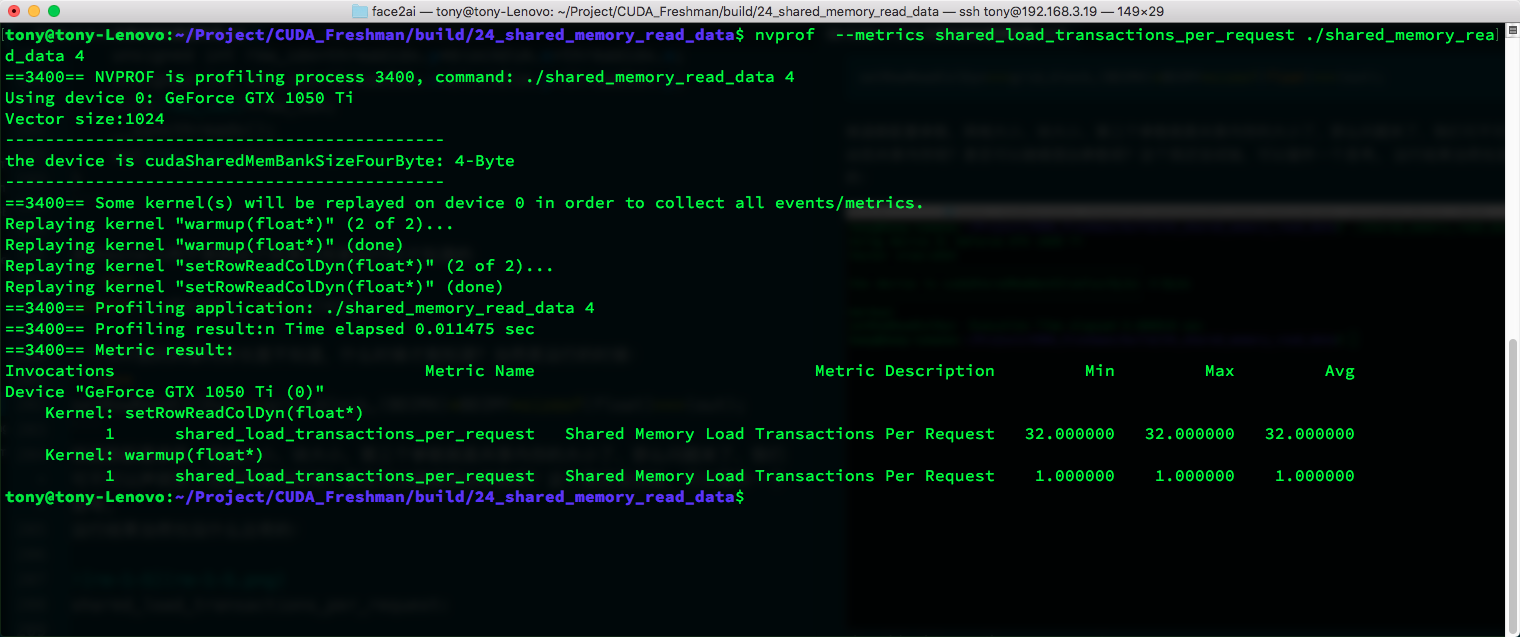shared_store_transactions_per_request: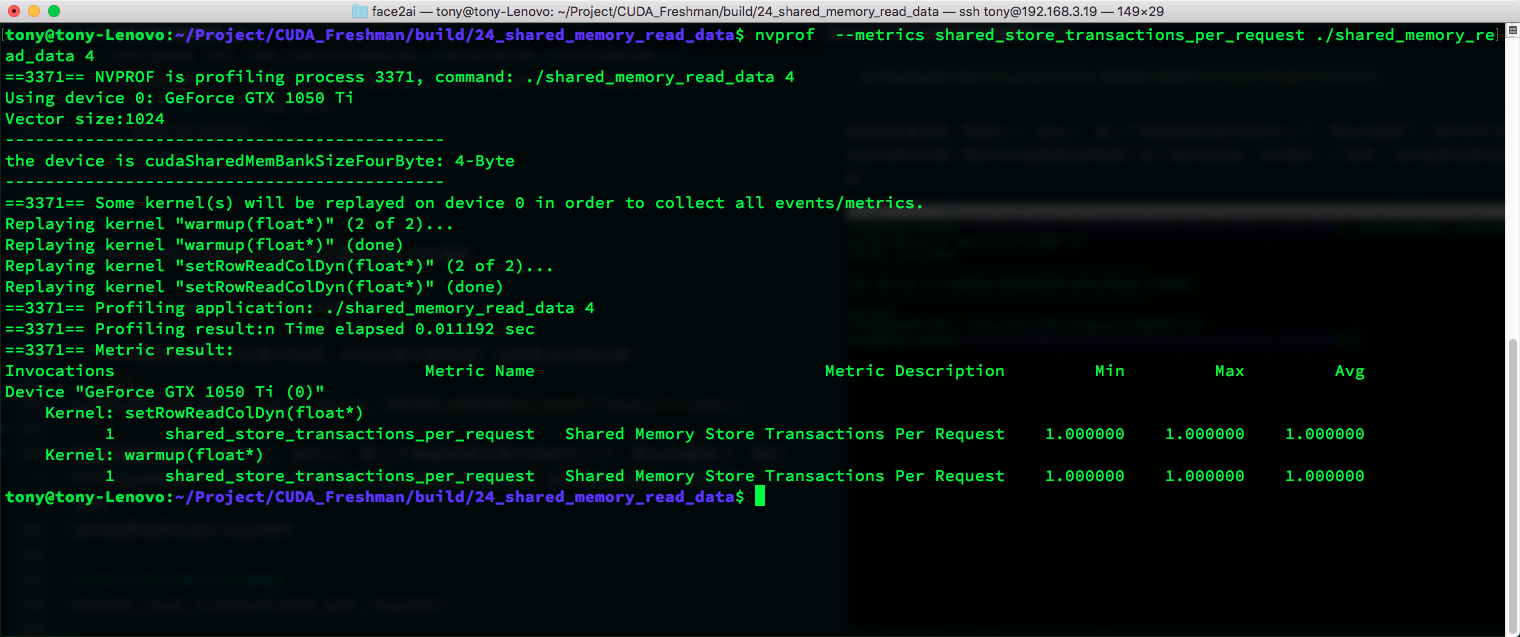### 填充静态声明的共享内存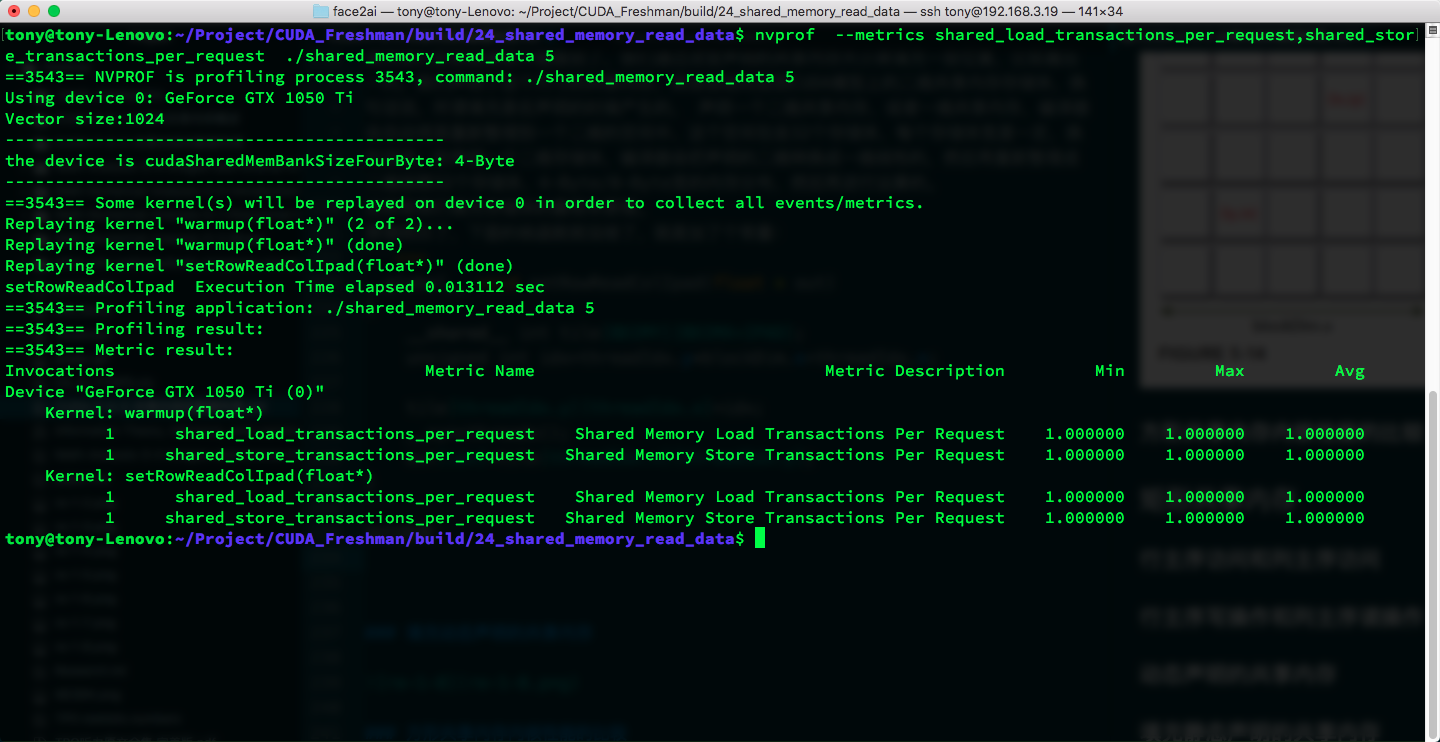### 填充动态声明的共享内存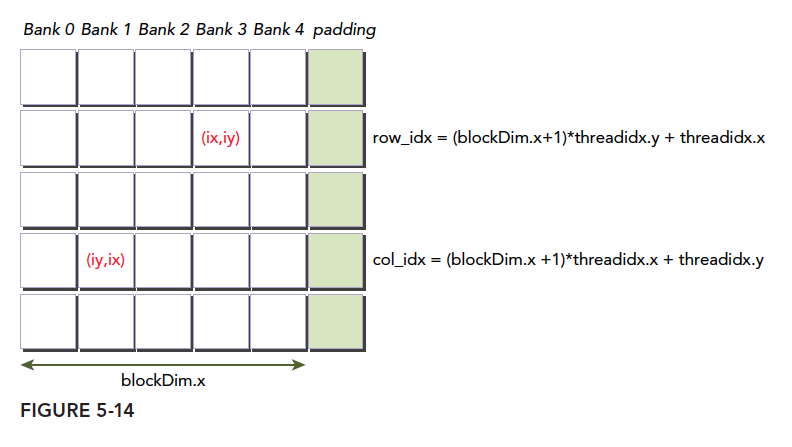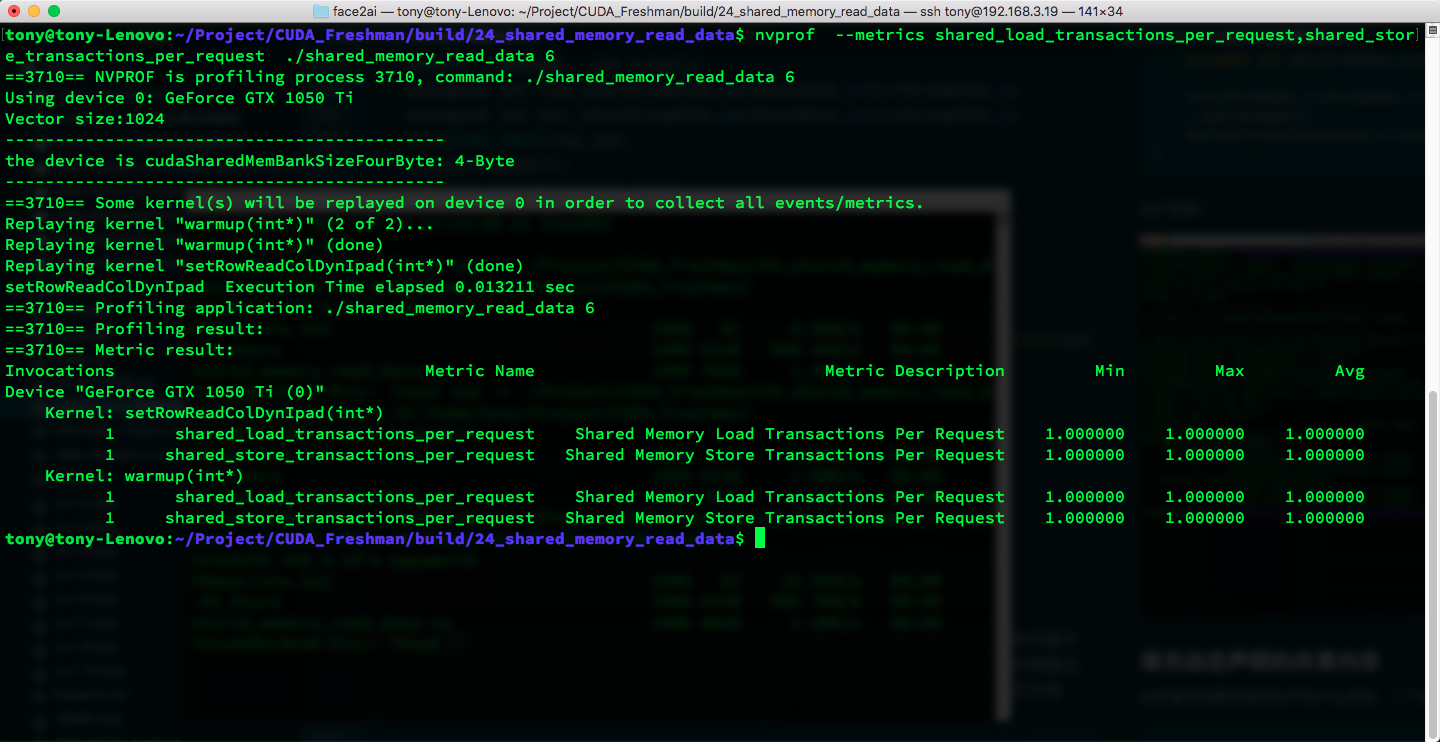### 方形共享内存内核性能的比较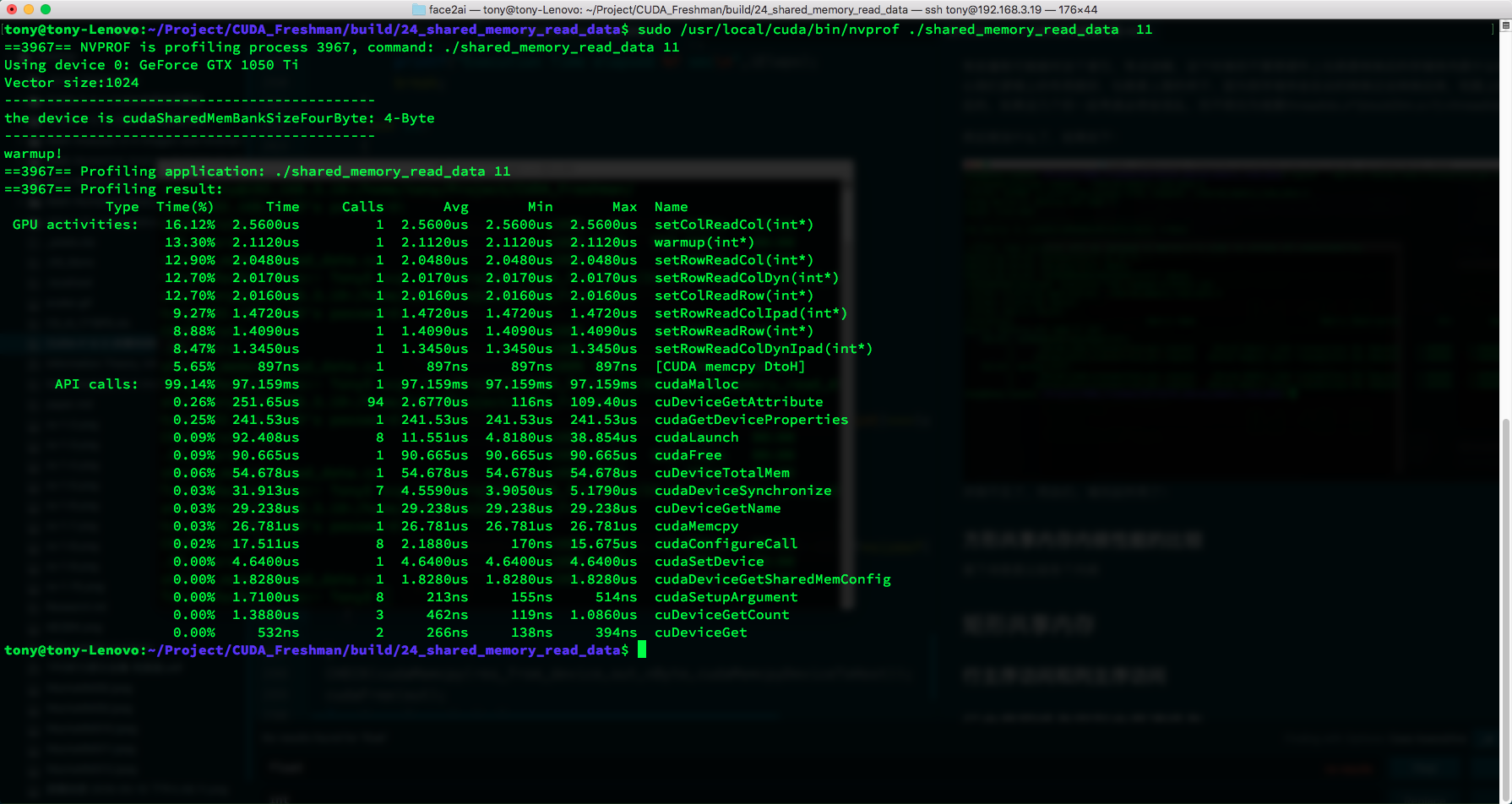Type Time(%) Time Calls Avg Min Max Name
GPU activities: 16.12% 2.5600 $\mu$ s 1 2.5600 $\mu$ s 2.5600 $\mu$ s 2.5600 $\mu$ s setColReadCol(int*)
13.30% 2.1120 $\mu$ s 1 2.1120 $\mu$ s 2.1120 $\mu$ s 2.1120 $\mu$ s warmup(int*)
12.90% 2.0480 $\mu$ s 1 2.0480 $\mu$ s 2.0480 $\mu$ s 2.0480 $\mu$ s setRowReadCol(int*)
12.70% 2.0170 $\mu$ s 1 2.0170 $\mu$ s 2.0170 $\mu$ s 2.0170 $\mu$ s setRowReadColDyn(int*)
12.70% 2.0160 $\mu$ s 1 2.0160 $\mu$ s 2.0160 $\mu$ s 2.0160 $\mu$ s setColReadRow(int*)
9.27% 1.4720 $\mu$ s 1 1.4720 $\mu$ s 1.4720 $\mu$ s 1.4720 $\mu$ s setRowReadColIpad(int*)
8.88% 1.4090 $\mu$ s 1 1.4090 $\mu$ s 1.4090 $\mu$ s 1.4090 $\mu$ s setRowReadRow(int*)
8.47% 1.3450 $\mu$ s 1 1.3450 $\mu$ s 1.3450 $\mu$ s 1.3450 $\mu$ s setRowReadColDynIpad(int*)

## 矩形共享内存

### 行主序写操作和列主序读操作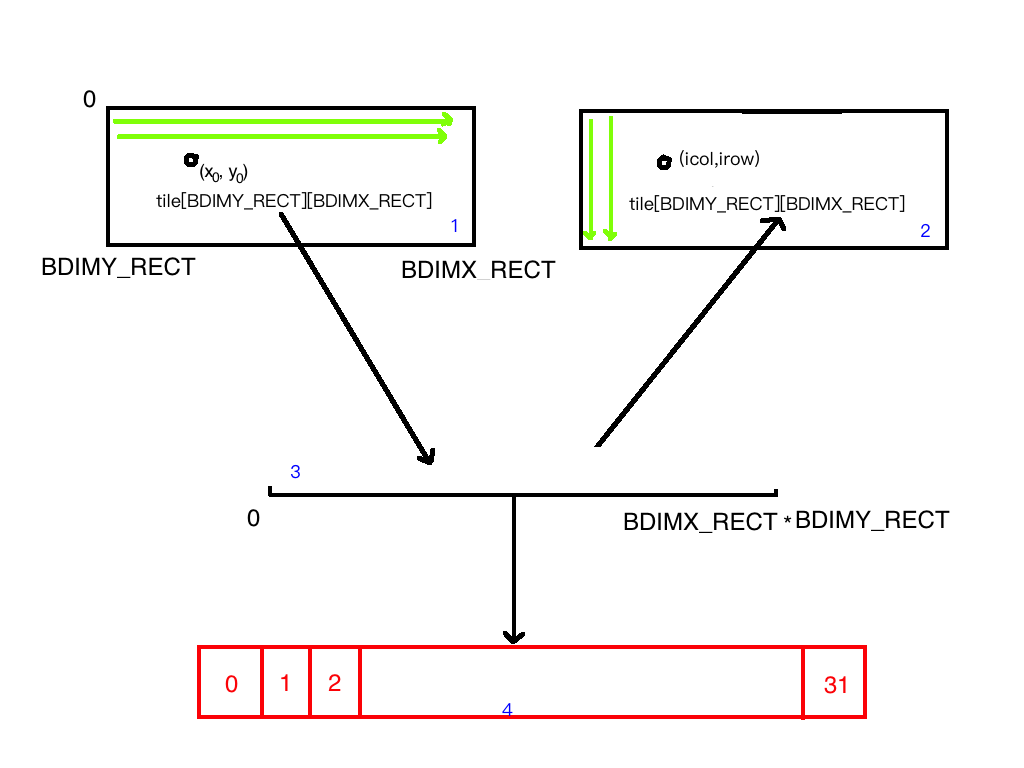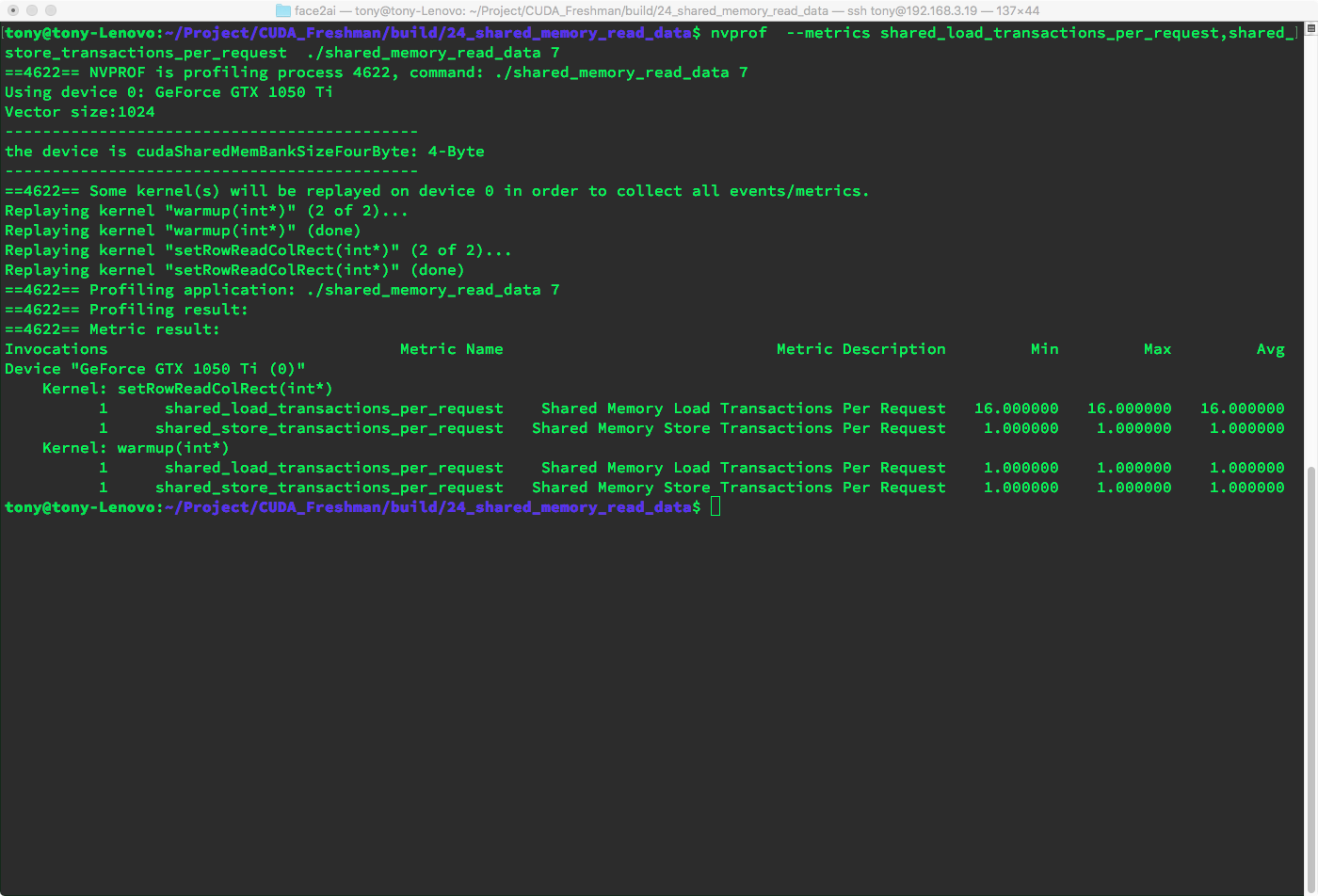### 动态声明的共享内存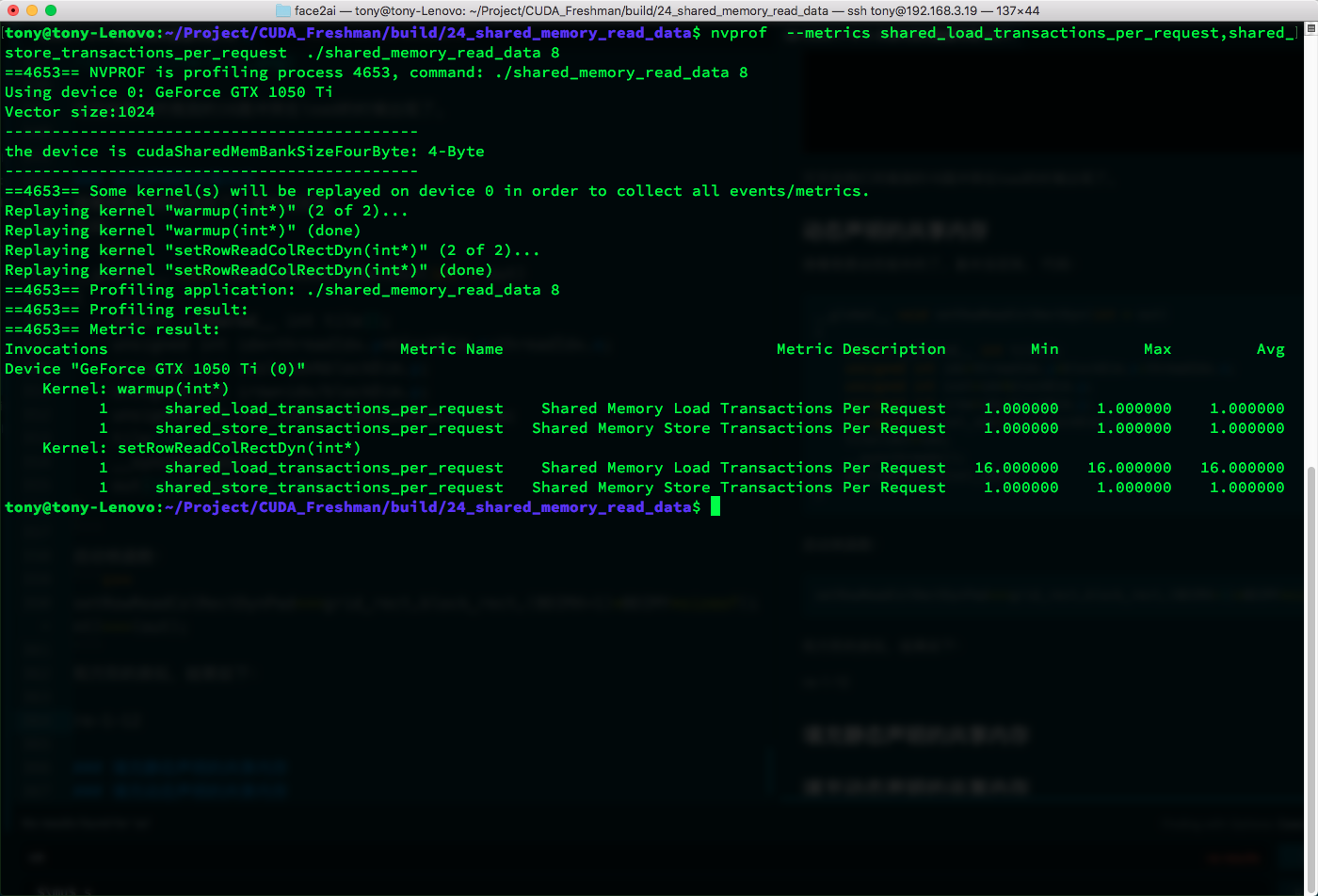### 填充静态声明的共享内存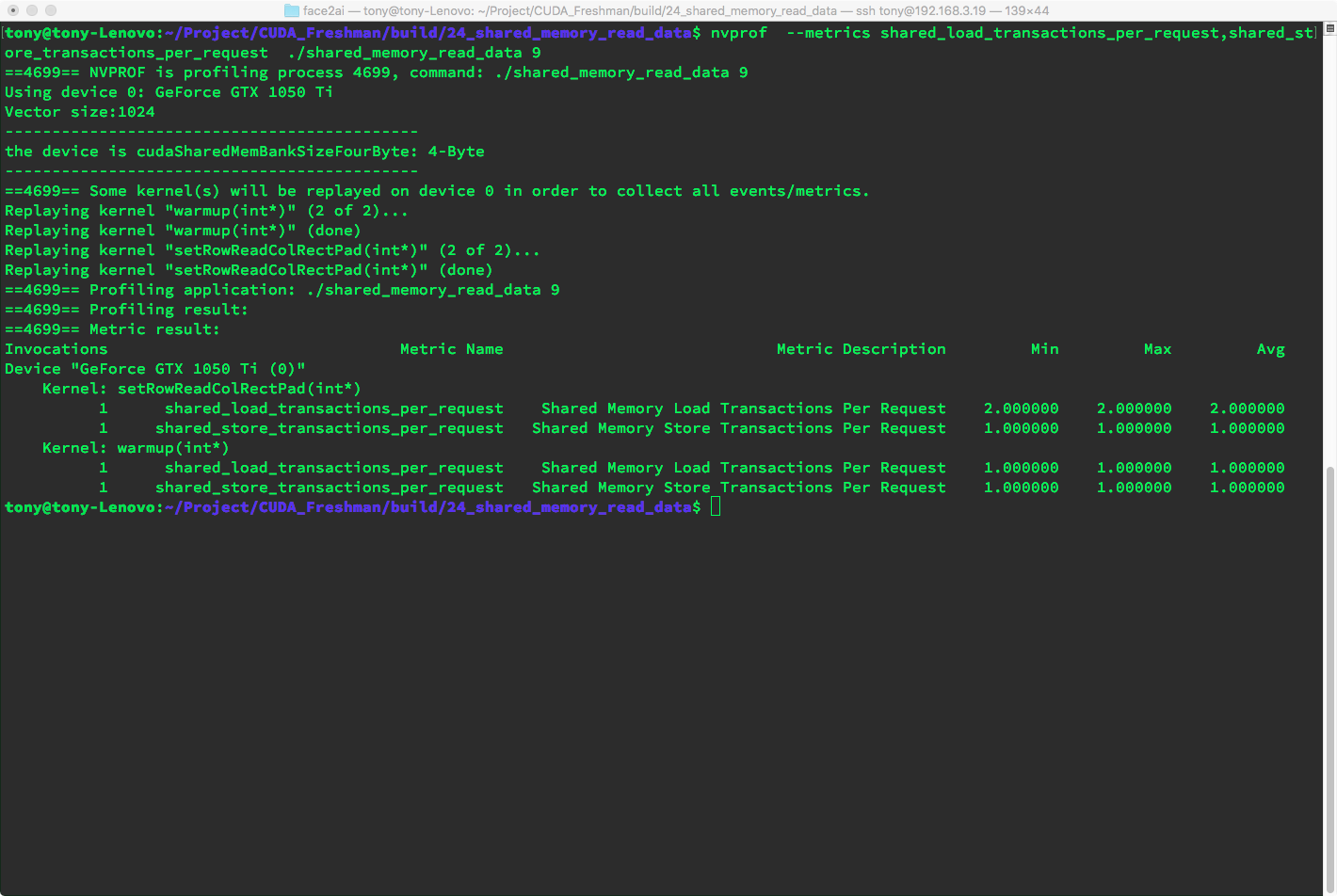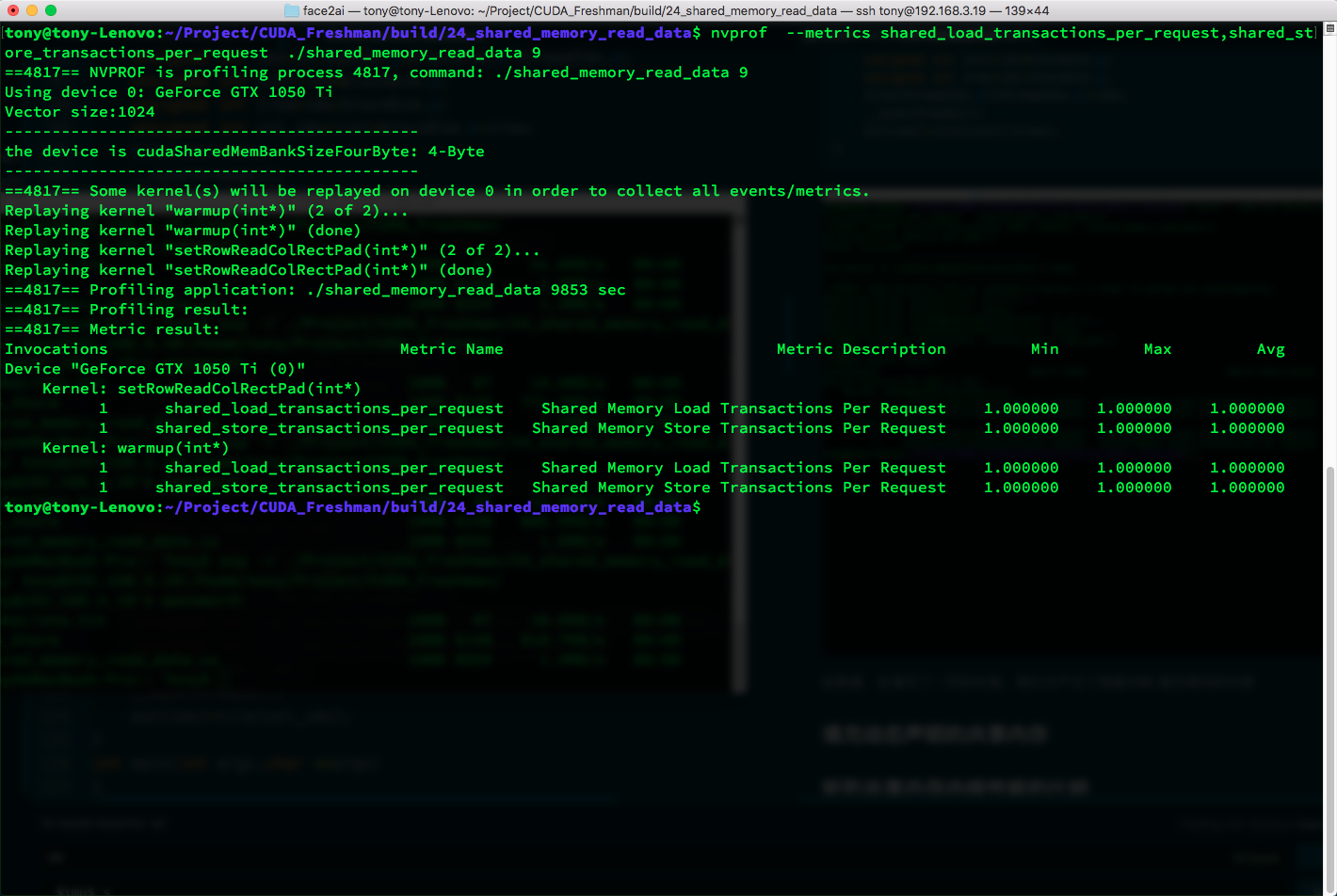### 填充动态声明的共享内存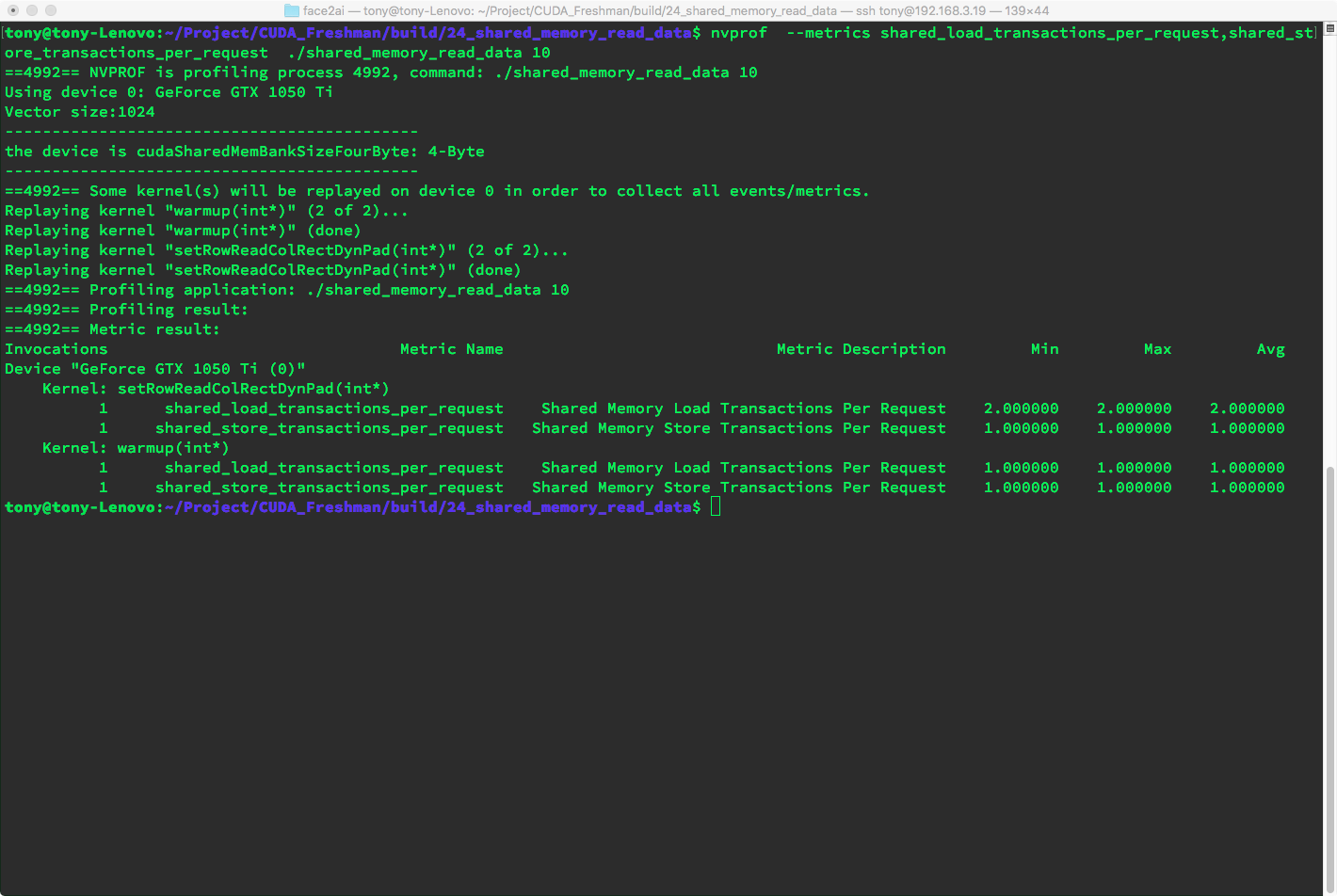### 矩形共享内存内核性能的比较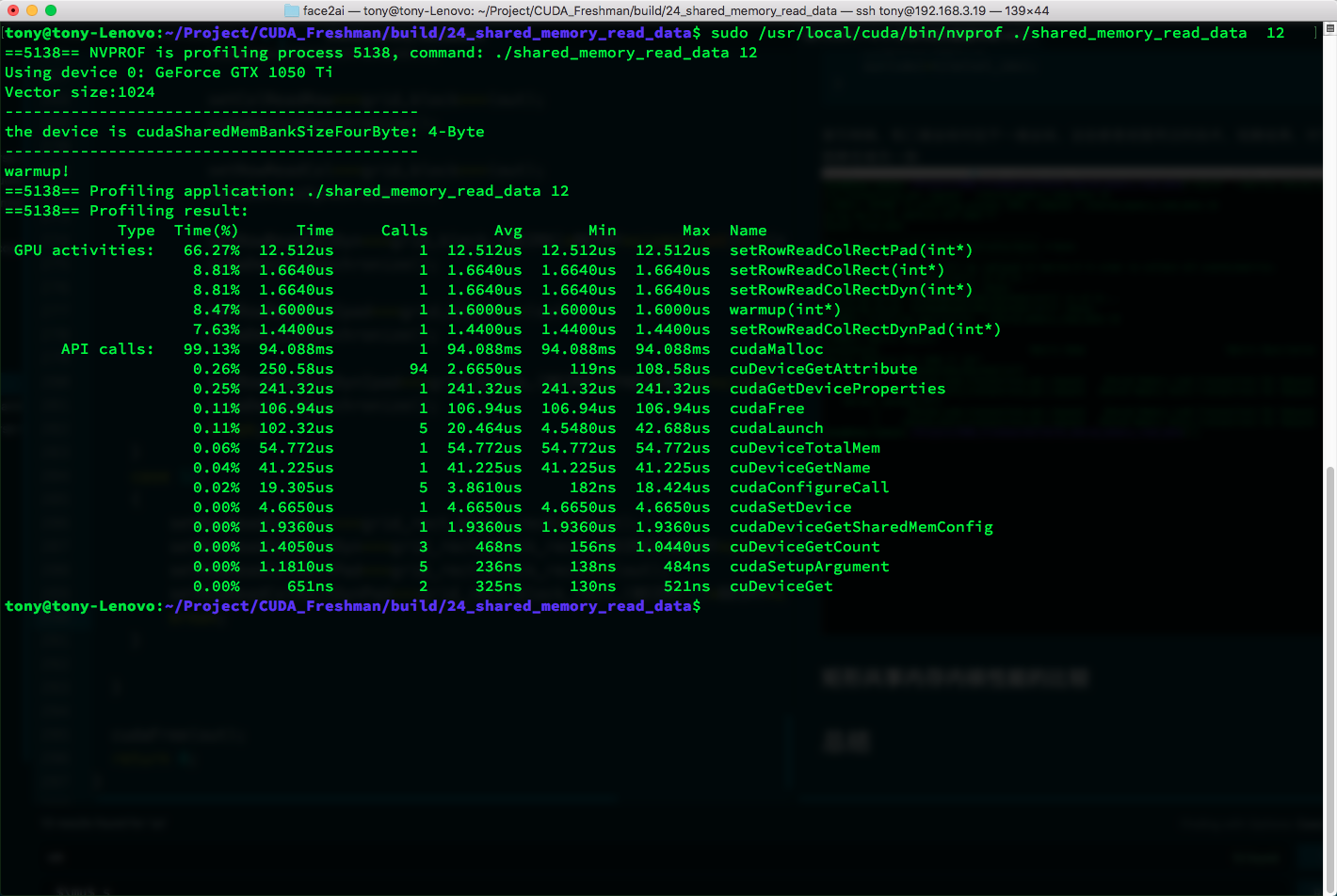Type Time(%) Time Calls Avg Min Max Name